# Extract Text After a Character in Excel (6 Ways)

In Microsoft Excel, extracting text from a cell is a crucial task. You may find yourself in many situations where you need to extract a  text after a specific character. You will learn effective and potential ways to extract text after a character in Excel from this tutorial. This tutorial will be on point with suitable examples and proper illustrations.

Extract Text After a Character.xlsm

## 6 Effective Ways to Extract Text After a Character in Excel

In the following section, we are providing you with six suitable and effective methods that you can implement into your dataset. Make sure you learn them all. We recommend you learn and apply all these. It will surely enrich your Excel knowledge.

### 1. Use MID and FIND Functions to Extract Text After a Character

Now, in this method, we are using the MID function and the FIND function together. We will find the specific character first from the cell using the FIND function. After that, we will extract the text from that particular position of that cell.

Take a look at the following dataset: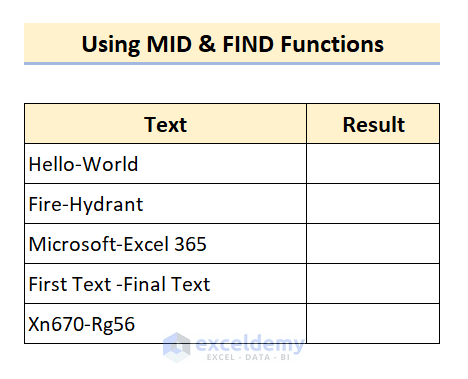Here, you can see we have some data in the dataset. All of the cells have the hyphen (“-”) in them. Now, our goal is to extract the text after the specific character hyphen (“-”) with our formula.

📌 Steps

• First, type the following formula in Cell C5:

`=MID(B5,FIND("-",B5)+1,LEN(B5))`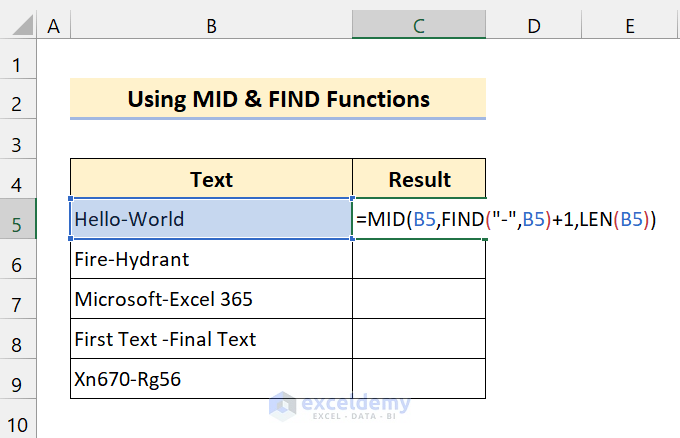Here we used the LEN function to provide several characters so that it can extract the rest of it.

• After that, press Enter.
• Now, drag the Fill handle icon over the range of cells C6:C9.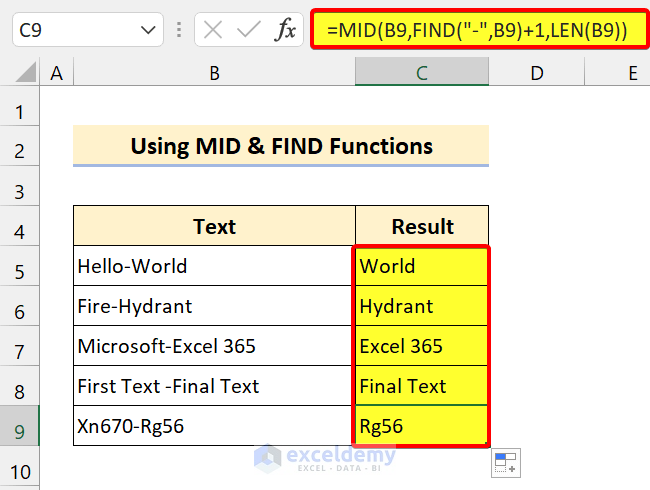As you can see, we are successful to extract text from a cell after a specific character in Excel. Give it a try now.

🔎 Breakdown of the Formula

Here, we are only breaking it down for the first data.

`➤` LEN(B5) returns 11.

`➤` FIND(“-“,B5) returns 6.

`➤` MID(B5,FIND(“-“,B5)+1,LEN(B5)) = MID(B5,6+1,11) returns World.

### 2. RIGHT, LEN, and FIND Functions to Extract Text After a Character

Now, in this method, we are using the RIGHT Functionthe LEN function, and the FIND function altogether to extract text from the cell. To demonstrate this, we are using the previous dataset.

Basically, we are extracting a substring from a cell after a specific character.

📌 Steps

• Now, type the following formula in Cell C5:

`=RIGHT(B5,LEN(B5)-FIND("-",B5))`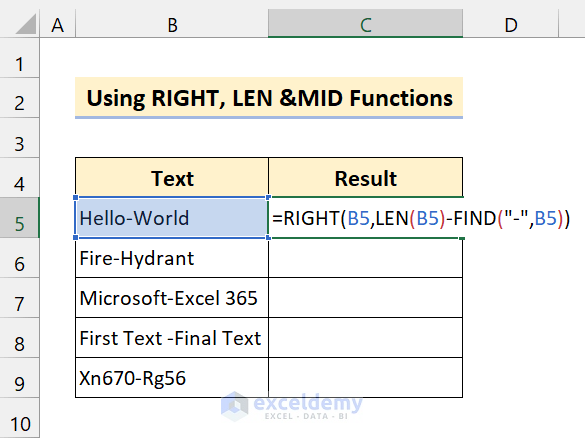• Then, press Enter.
• After that, drag the Fill handle icon over the range of cells C6:C9.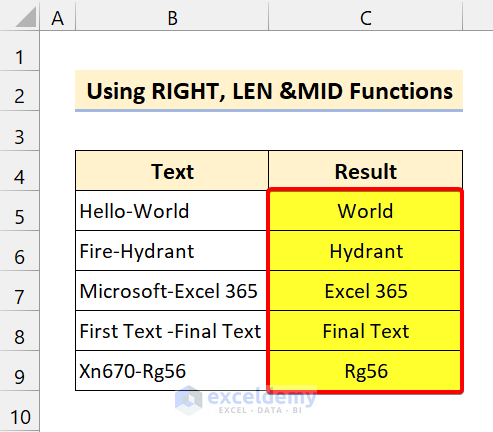As you can see, we have successfully found the specific character from a specific position. And we extract the text after that particular character from the cell.

🔎 Breakdown of the Formula

Here, we are only breaking it down for the first data.

`➤` LEN(B5) returns 11.

`➤` FIND(“-“,B5) returns 6.

`➤` RIGHT(B5,LEN(B5)-FIND(“-“,B5)) =RIGHT(B5,11-6)  returns World.

### 3. Use of LEFT, FIND, and SUBSTITUTE Functions to Excerpt Text After a Character

Now, we are using the LEFT functionthe FIND function, and the SUBSTITUTE function of Excel. Take a look at the following dataset: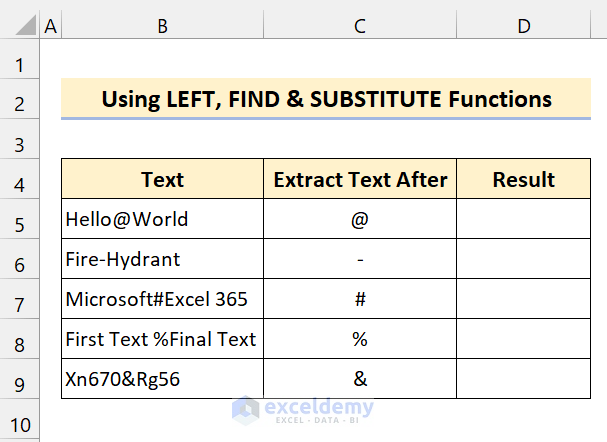Now, this dataset is pretty interesting. We are using the previous dataset. But, we changed the characters. We have multiple characters in the cells. Here, our goal is to extract the text from the cells after all those particular characters using our formula.

📌 Steps

• At first, type the following formula in Cell D5:

`=SUBSTITUTE(B5,LEFT(B5,FIND(C5,B5)),"")`

• Now, press Enter.
• Then, drag the Fill handle icon over the range of cells D6:D9.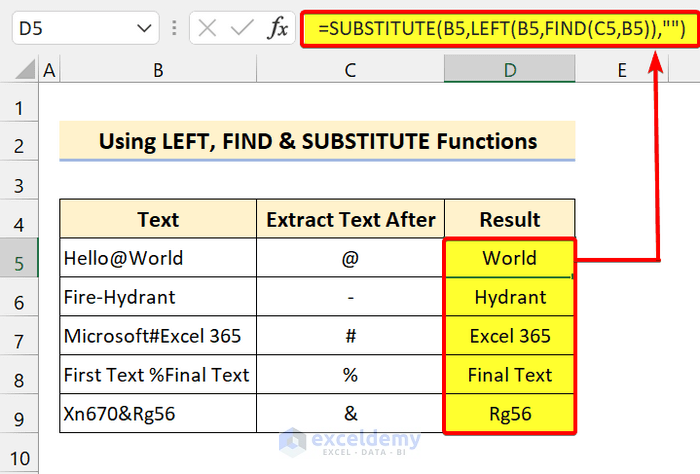As you can see, our formula worked pretty well to extract text after those individual characters in Excel.

🔎 Breakdown of the Formula

Here, we are only breaking it down for the first data.

`➤` FIND(C5,B5) returns 6.

`➤` LEFT(B5,6) returns [email protected]

`➤` SUBSTITUTE(B5,LEFT(B5,FIND(C5,B5)),””) = SUBSTITUTE(B5,”[email protected]”,””)  returns World.

Read More: How to Extract Text after a Specific Text in Excel (10 Ways)

### 4. Using RIGHT, SEARCH, and SUBSTITUTE Functions to Extract Specific Characters

Now, this formula is pretty complex. But, if you have an idea about these functions, you will get this easily. To perform this, we are using the following functions:

1. The RIGHT function.
2. The SEARCH function.
3. The SUBSTITUTE function.
4. The LEN function.

To demonstrate this method, we are using the previous dataset here. Make sure you check all these functions before getting into it.

📌 Steps

• First, type the following formula in Cell D5:

`=RIGHT(B5,LEN(B5)-SEARCH("#",SUBSTITUTE(B5,C5,"#",LEN(B5)-LEN(SUBSTITUTE(B5,C5,"")))))`

• After that, press Enter.
• Now, drag the Fill handle icon over the range of cells D6:D9.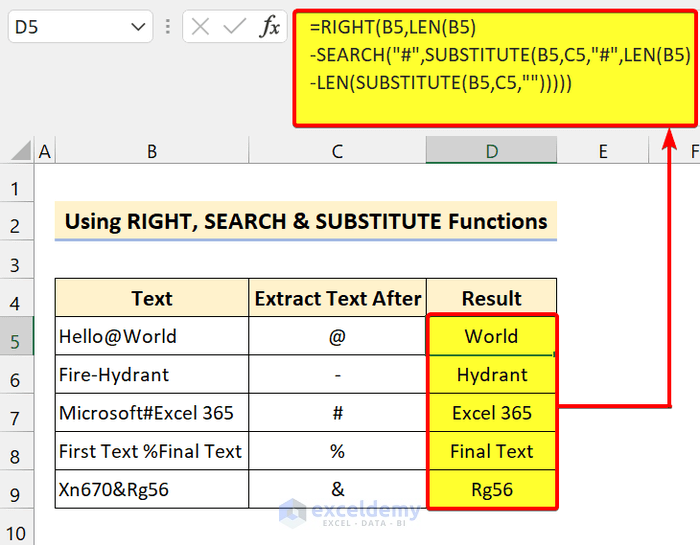In the end, we successfully selected the specific characters and their positions. After that, we extracted the text after the specific character.

🔎 Breakdown of the Formula

Here, we are only breaking it down for the first data.

`➤` LEN(B5) returns 11

`➤` SUBSTITUTE(B5,C5,””) returns HelloWorld.

`➤` SUBSTITUTE(B5,C5,”#”,11-LEN(“HelloWorld”)) returns Hello#World.

`➤` SEARCH(“#”,”Hello#World”) returns 6.

`➤` RIGHT(B5,LEN(B5)-SEARCH(“#”,SUBSTITUTE(B5,C5,”#”,LEN(B5)-LEN(SUBSTITUTE(B5,C5,””))))) = RIGHT(B5,11-6)  returns World.

Read More: How to Extract Text Between Two Characters in Excel (4 Methods)

### 5. Using RIGHT, SUBSTITUTE, and REPT Functions to Extract Text After a Character

Here, our formula consists of multiple functions of Excel. Our main three functions to extract text are the RIGHT functionthe SUBSTITUTE functions, and the REPT function.

To demonstrate this, we are using a similar dataset to the previous one.

📌 Steps

• At first, type the following formula in Cell D5:

`=TRIM(RIGHT(SUBSTITUTE(B5,C5,REPT(" ",LEN(B5))),LEN(B5)))`

We used the TRIM function to remove extra leading spaces.

• After that, press Enter.
• Now, drag the Fill handle icon over the range of cells D6:D9.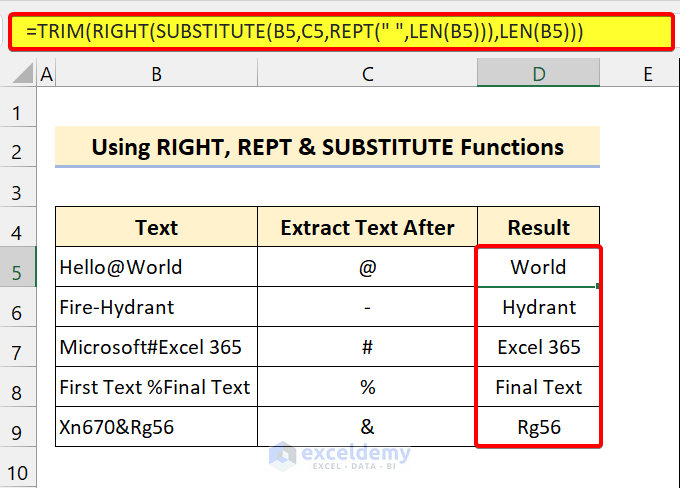As you can see, we are successful to extract text after a character from an Excel cell. Try this method with different characters from different positions.

🔎 Breakdown of the Formula

Here, we are only breaking it down for the first data.

`➤` LEN(B5) returns 11

`➤` REPT(” “,LEN(B5)) returns “           “ (Spaces).

`➤` SUBSTITUTE(B5,C5,REPT(” “,LEN(B5))) returns “Hello           World”.

`➤` RIGHT(SUBSTITUTE(B5,C5,REPT(” “,LEN(B5))),LEN(B5)) returns  “     World”.

`➤` TRIM(RIGHT(SUBSTITUTE(B5,C5,REPT(” “,LEN(B5))),LEN(B5))) = TRIM(”      World”) returns World.

Read More: How to Extract Text After Last Space in Excel (5 Ways)

### 6. VBA Codes to Extract Text After a Character in Excel

If you are a VBA freak like me, you can try this method. This code will extract text after a character at ease. With this simple code, you will be able to perform this operation for an entire column.

📌 Steps

• First, press Alt+F11 on your keyboard to open the VBA editor.
• Then, select Insert > Module.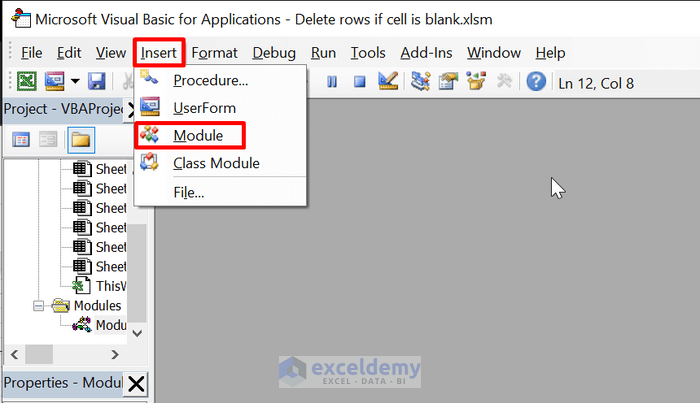• After that, type the following code:
``````Sub extract_text()

Dim rng As Range
Dim cell As Range
Set rng = Application.Selection
For Each cell In rng
cell.Offset(0, 1).Value = Right(cell, Len(cell) - InStr(cell, "-"))
Next cell

End Sub``````
• Then, save the file.
• Now, select the range of cells B5:B9.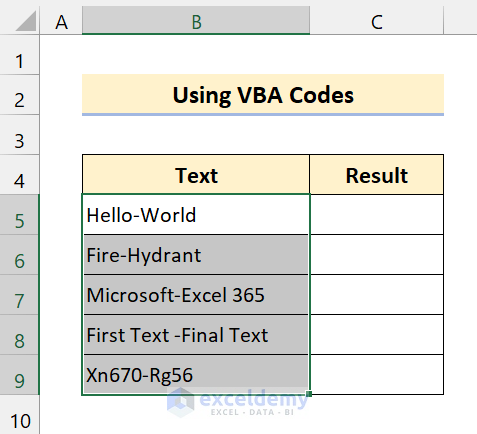• After that, press Alt+F8 on your keyboard to open the Macro dialog box.
• Next, select extract_text.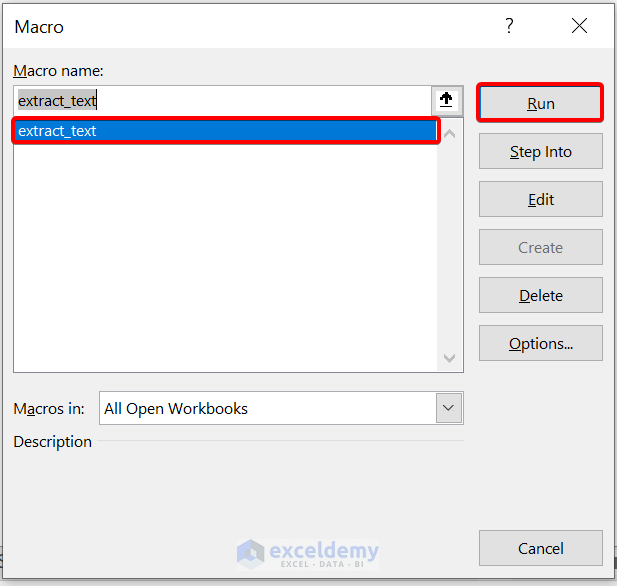• Then, click on Run.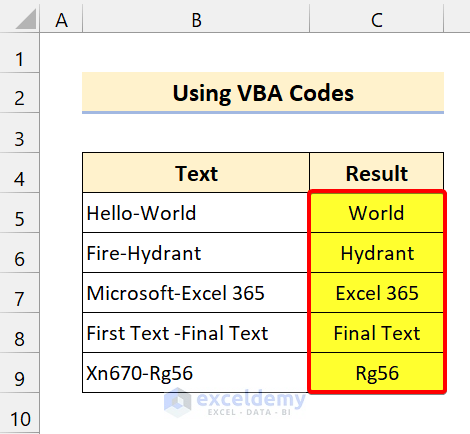Here, by using the VBA code, we successfully extracted the text after the specific character.

Read More: How to Extract Certain Text from a Cell in Excel VBA (5 Examples)

## 💬 Things to Remember

✎ Before you perform these, make sure to check the links to know the functions we used.

✎ If you see any #VALUE! Error, wrap the whole formula under the IFERROR function.

## Conclusion

To conclude, I hope this tutorial has provided you with a piece of useful knowledge to extract text after a specific character in Excel. We recommend you learn and apply all these instructions to your dataset. Download the practice workbook and try these yourself. Also, feel free to give feedback in the comment section. Your valuable feedback keeps us motivated to create tutorials like this.

Don’t forget to check our website Exceldemy.com for various Excel-related problems and solutions.

Keep learning new methods and keep growing!

## Related Articles#### Pabok

We will be happy to hear your thoughtsEnable registration in settings - general
• Total (0)
0